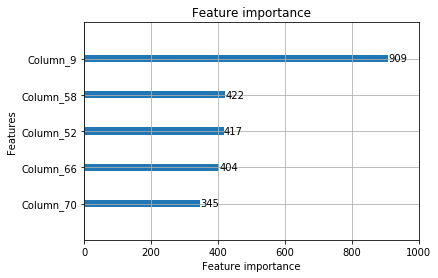# Wildfire AWARE: Machine Learning using Gradient Boosted Decision Trees.¶

In this document we provide an overview of our model and the thought process behind it. This includes briefly touching upon the data we used and providing a visual depiction of what our trained model values.

Let us begin by showing some high level details about the data we have been working with.

In :
import sqlite3
import pandas as pd
import numpy as np

In :
def dataOverview():
"""Load data and handle Nans from csv into data frame
"""

data_file = './data/fireData.sqlite'
conn = sqlite3.connect(data_file)
df = pd.read_sql('SELECT * FROM weatherData', conn)
conn.close()
for name in df.columns[0:-1]:
if('PRECIP_INTENSITY' != name):
df[name].replace('0', np.nan, inplace=True)

#drop rows with Na's. This would significantly reduce the dataset size.
#df.dropna(subset=['PRESSURE','WIND_BEARING','WIND_SPEED','DEW_POINT','HUMIDITY','DAY_TIME_TEMP','FULL_DAY_TEMP','DAY_TIME_WINDGUST','FULL_DAY_WINDGUST'],inplace=True)

#cols = ['LATITUDE','LONGITUDE','FIRE_YEAR','FIRE_DATE','FIRE_SIZE','FIRE_SIZE_CLASS','FIRE_CAUSE_CODE','ELEVATION','UV_INDEX','PRECIP_ACCUMULATION','PRECIP_TYPE']

#print('\n {}'.format(df.shape))
return df

def missing_values_table(df):
"""Calculate missing values by column, tabulate results

Input
df: The dataframe
"""
# Total missing values
mis_val = df.isnull().sum()

# Percentage of missing values
mis_val_percent = 100 * df.isnull().sum() / len(df)

# Make a table with the results
mis_val_table = pd.concat([mis_val, mis_val_percent], axis=1)

# Rename the columns
mis_val_table_ren_columns = mis_val_table.rename(
columns = {0 : 'Missing Values', 1 : '% of Total Values'})

# Sort the table by percentage of missing descending
mis_val_table_ren_columns = mis_val_table_ren_columns[
mis_val_table_ren_columns.iloc[:,1] != 0].sort_values(
'% of Total Values', ascending=False).round(1)

# Print some summary information
print ("Your selected dataframe has " + str(df.shape) + " columns.\n"
"There are " + str(mis_val_table_ren_columns.shape) +
" columns that have missing values.")

# Return the dataframe with missing information
return mis_val_table_ren_columns

In :
df = dataOverview()

    LATITUDE   LONGITUDE  FIRE_YEAR     FIRE_DATE FIRE_SIZE FIRE_SIZE_CLASS  \
0  31.883333 -110.023333     1993.0  7.388064e+08       0.5               B
1  31.883333 -110.023333     1993.0  7.455456e+08       0.5               B
2  31.883333 -110.023333     1993.0  7.312032e+08       0.5               B
3  31.883333 -110.023333     1993.0  7.431264e+08       0.5               B
4  34.536227  -88.521878     2010.0  1.267834e+09      50.0               C

FIRE_CAUSE_CODE  ELEVATION       DAY_TIME_TEMP       FULL_DAY_TEMP  \
0             4.0        NaN  26.552857142857142  23.918750000000003
1             4.0        NaN  25.786428571428576   23.18541666666667
2             4.0        NaN  12.508333333333335   9.777916666666666
3             4.0        NaN  28.434285714285714  25.909166666666668
4            13.0        NaN   8.793636363636365   4.277500000000001

...          WIND_SPEED WIND_BEARING PRECIP_INTENSITY PRECIP_TYPE  \
0         ...                2.39          122           0.0686        rain
1         ...                1.73          231           0.1727        rain
2         ...                1.94          291           0.1194        rain
3         ...                1.02          110           0.1473        rain
4         ...                0.93           17                0    NOPrecip

PRECIP_ACCUMULATION PRESSURE UV_INDEX CLOUD_COVER DEW_POINT  \
0                 NaN  1010.47      NaN        0.12     -2.36
1                 NaN  1012.02      NaN        0.58     13.99
2                 NaN  1015.29      NaN        0.12     -1.59
3                 NaN  1011.54      NaN         0.3     12.28
4                 NaN  1024.43      NaN         NaN     -5.56

WILDFIRE_OCCURRENCE
0                  1.0
1                  0.0
2                  0.0
3                  0.0
4                  1.0

[5 rows x 23 columns]


Above we are showing the first five values for each column in our dataset. as shown above our job was to work with 23 different features. Lets determine the distribution of NaNs.

In :
missing_values = missing_values_table(df)

Your selected dataframe has 23 columns.
There are 13 columns that have missing values.
Missing Values  % of Total Values
ELEVATION                    399092              100.0
UV_INDEX                     399092              100.0
PRECIP_ACCUMULATION          377846               94.7
CLOUD_COVER                   35550                8.9
PRESSURE                       5282                1.3
WIND_BEARING                   2414                0.6
WIND_SPEED                     1589                0.4
DEW_POINT                      1457                0.4
HUMIDITY                       1403                0.4
DAY_TIME_TEMP                  1275                0.3
FULL_DAY_TEMP                  1275                0.3
DAY_TIME_WINDGUST              1275                0.3
FULL_DAY_WINDGUST              1275                0.3


Evidently we have some redundant features. We removed ELEVATION and UV_INDEX going forward. In the future we would like to obtain this data so that we can consider it.

We constructed additional features that were used in training given these features as primitives. We found a 5% increase in AUC when doing so. Ultimately we obtained a 3 fold cross validated AUC of 0.75.

After some deliberation we opted to use gradient boosted decision trees for our production model. This decision was motivated by their effectiveness in our empirical tests.

This model works by additively combining weak learners in the form of decision trees 1. The idea is that by combining a set of 'rules of thumb' one can construct a powerful inference technique.The code below shows how easy it is to load in a trained Gradient boosted decision trees model. It demonstrates loading in the wildfire AWARE inference model and using it to determine the 5 most important features.

In :
import lightgbm as lgb
import matplotlib.pyplot as plt

"""
gbm = lgb.Booster(model_file=location)

return gbm


Plot feature importances...